# 如何在 Apache Flink 1.10 中使用 Python UDF?

+关注继续查看

## PyFlink 支持 Python UDFs 的架构

Apache Beam 是一个统一编程模型框架，实现了可使用任何语言开发可以运行在任何执行引擎上的批处理和流处理作业，这得益于 Beam 的 Portability Framework，如下图所示：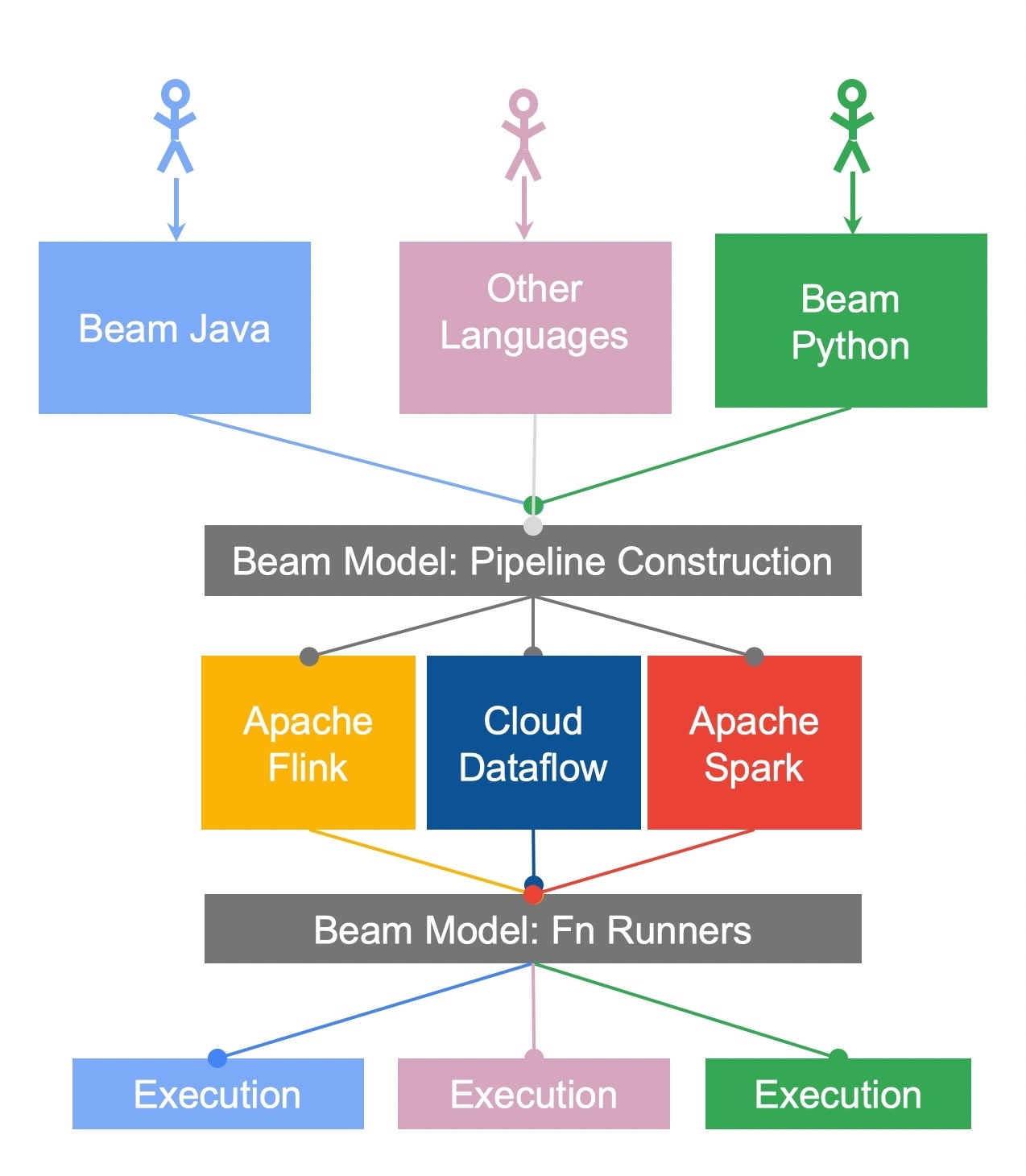Portability Framework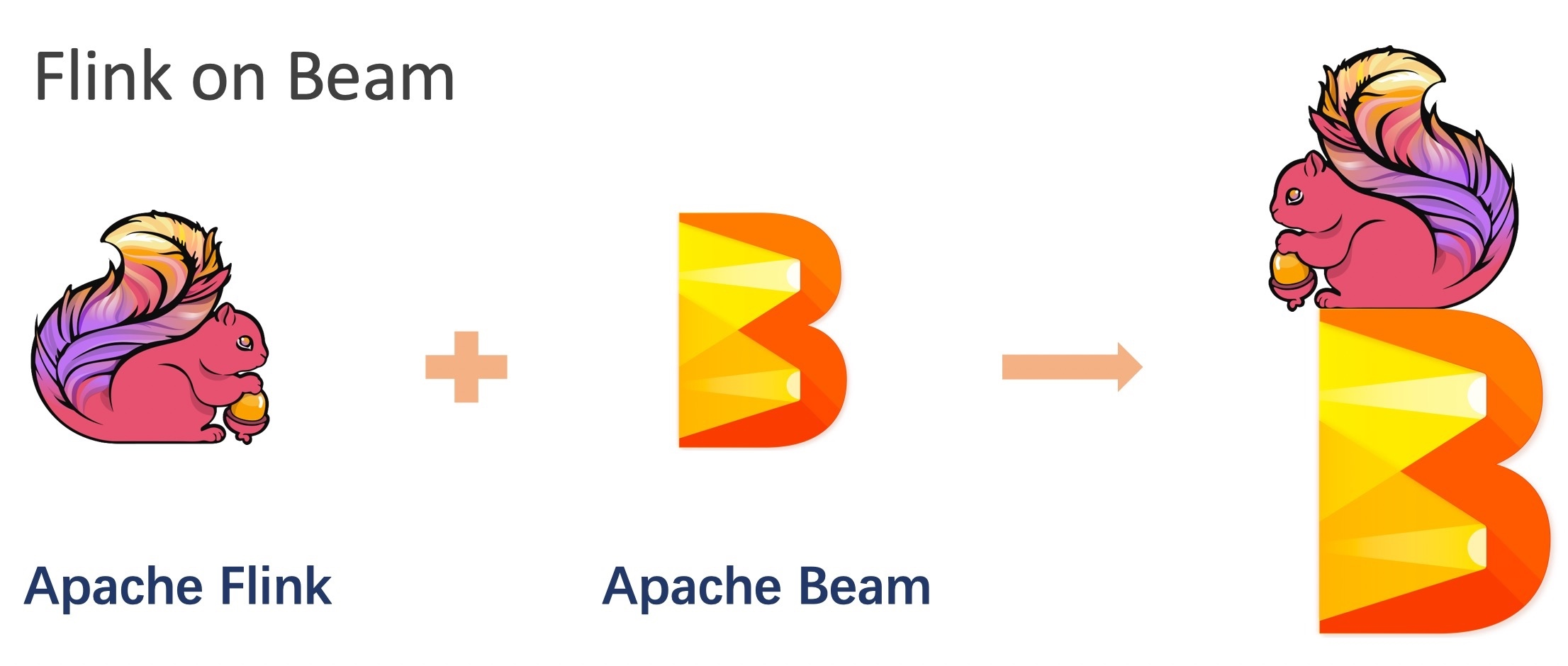PyFlink 对 Python UDFs 的支持上，Python 的运行环境管理以及 Python 运行环境 Python VM 和 Java 运行环境 JVM 的通讯至关重要。幸运的是，Apache Beam 的 Portability Framework 完美解决了这个问题。所以才有了如下 PyFlink on Beam Portability Framework 的架构如下：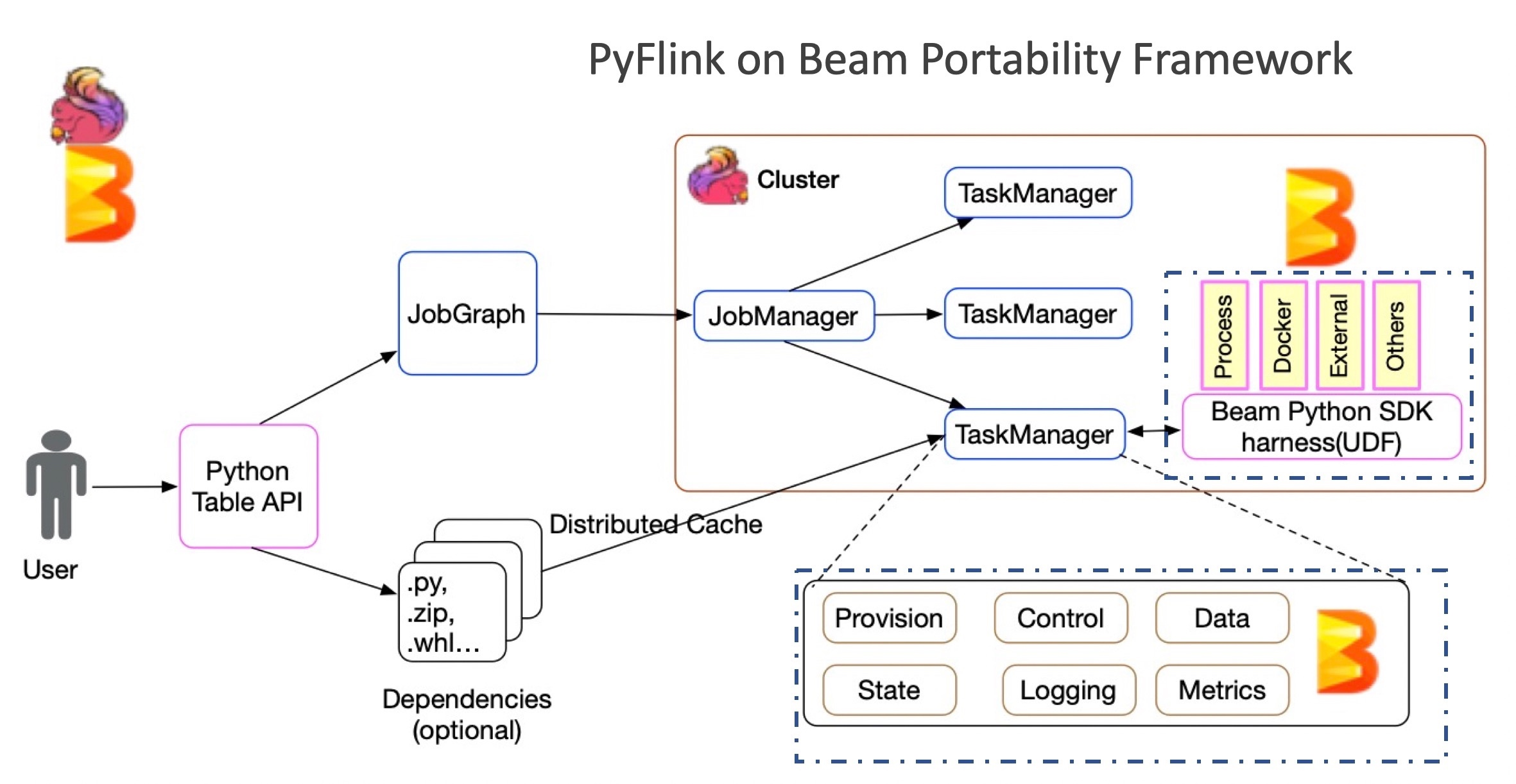Beam Portability Framework 是一个成熟的多语言支持框架，框架高度抽象了语言之间的通信协议(gRPC),定义了数据的传输格式(Protobuf)，并且根据通用流计算框架所需要的组件，抽象个各种服务，比如, DataService，StateService，MetricsService 等。

### JVM 和 Python VM 的通讯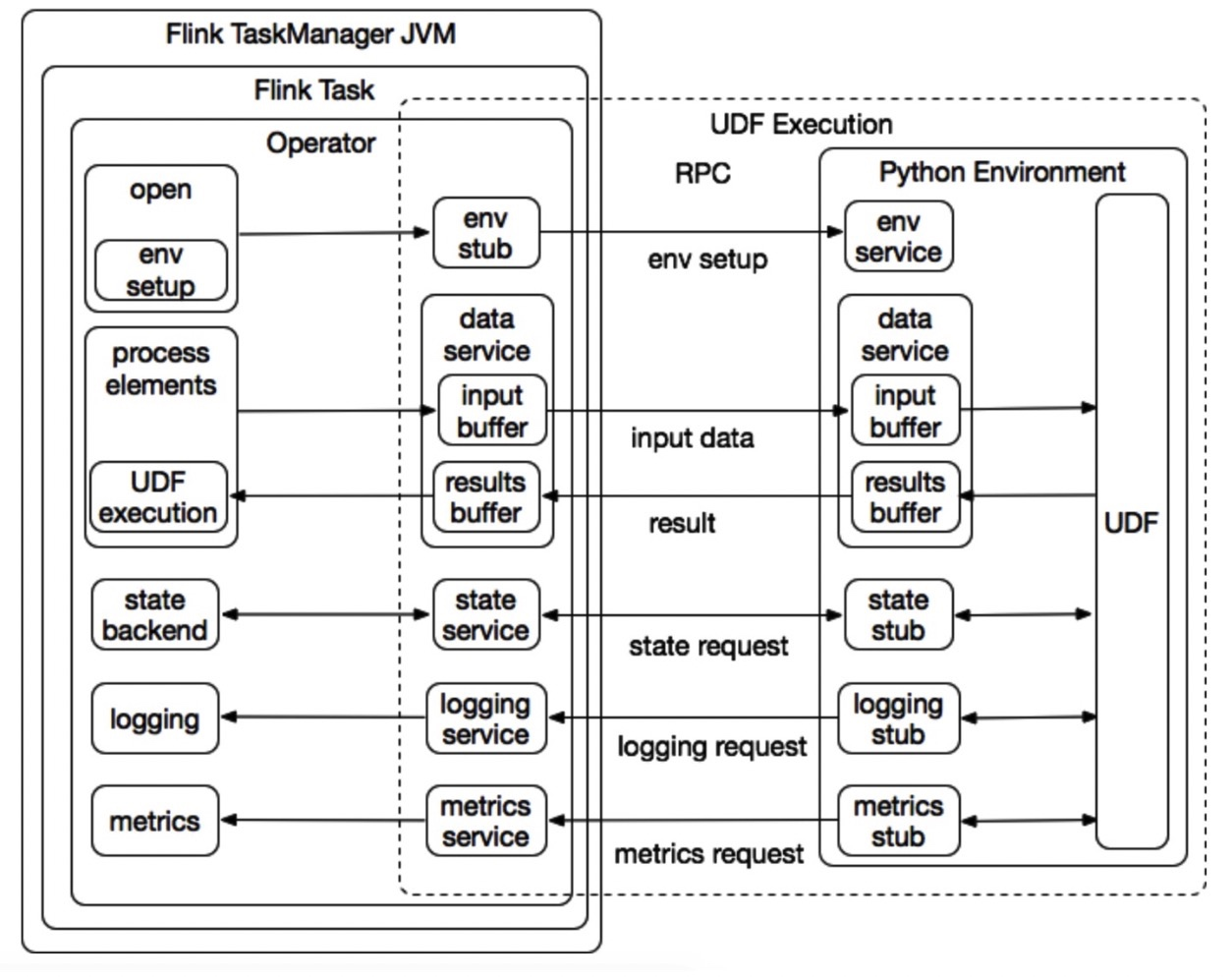Communication between JVM and Python VM

• 环境管理服务: 负责启动和终止 Python 执行环境。
• 数据服务: 负责在 Apache Flink 算子和 Python 执行环境之间传输输入数据和接收用户 UDF 的执行结果。
• 日志服务: 是记录对用户 UDF 日志输出支持的机制。它可以将用户 UDF 产生的日志传输到 Apache Flink 算子，并与 Apache Flink 的日志系统集成。High-level flow between Python VM and JVM

• 初始化 Python 执行环境。

• Python UDF Runner 启动所需的 gRPC 服务，如数据服务、日志服务等。
• Python UDF Runner 另起进程并启动 Python 执行环境。
• Python worker 向 PythonUserDefinedFunctionRunner 进行注册。
• Python UDF Runner 向 Python worker 发送需要在 Python 进程中执行的用户定义函数。
• Python worker 将用户定义的函数转换为 Beam 执行算子（注意：目前，PyFlink 利用 Beam 的可移植性框架来执行 Python UDF）。
• Python worker 和 Flink Operator 之间建立 gRPC 连接，如数据连接、日志连接等。
• 处理输入元素。

• Python UDF Runner 通过 gRPC 数据服务将输入元素发送给 Python worker 执行。
• Python 用户定义函数还可以在执行期间通过 gRPC 日志服务和 metrics 服务将日志和 metrics 收集到 Python UDF Runner。
• 执行结果可以通过 gRPC 数据服务发送到 Python UDF Runner。

$python -m pip install apache-Apache Flink ### 定义一个 UDF 除了扩展基类 ScalarFunction 之外，定义 Python UDF 的方法有很多。下面的示例显示了定义 Python UDF 的不同方法，该函数以 BIGINT 类型的两列作为输入参数，并返回它们的和作为结果。 • Option 1: extending the base class ScalarFunction class Add(ScalarFunction): def eval(self, i, j): return i + j add = udf(Add(), [DataTypes.BIGINT(), DataTypes.BIGINT()], DataTypes.BIGINT()) • Option 2: Python function @udf(input_types=[DataTypes.BIGINT(), DataTypes.BIGINT()], result_type=DataTypes.BIGINT()) def add(i, j): return i + j • Option 3: lambda function add = udf(lambda i, j: i + j, [DataTypes.BIGINT(), DataTypes.BIGINT()], DataTypes.BIGINT()) • Option 4: callable function class CallableAdd(object): def __call__(self, i, j): return i + j add = udf(CallableAdd(), [DataTypes.BIGINT(), DataTypes.BIGINT()], DataTypes.BIGINT()) • Option 5: partial function return i + j + k add = udf(functools.partial(partial_add, k=1), [DataTypes.BIGINT(), DataTypes.BIGINT()], DataTypes.BIGINT()) ### 注册一个UDF • register the Python function table_env.register_function("add", add) • Invoke a Python UDF my_table.select(js "add(a, b)") • Example Code 下面是一个使用 Python UDF 的完整示例。 from PyFlink.table import StreamTableEnvironment, DataTypes from PyFlink.table.descriptors import Schema, OldCsv, FileSystem from PyFlink.table.udf import udf env = StreamExecutionEnvironment.get_execution_environment() env.set_parallelism(1) t_env = StreamTableEnvironment.create(env) t_env.register_function("add", udf(lambda i, j: i + j, [DataTypes.BIGINT(), DataTypes.BIGINT()], DataTypes.BIGINT())) t_env.connect(FileSystem().path('/tmp/input')) \ .with_format(OldCsv() .field('a', DataTypes.BIGINT()) .field('b', DataTypes.BIGINT())) \ .with_schema(Schema() .field('a', DataTypes.BIGINT()) .field('b', DataTypes.BIGINT())) \ .create_temporary_table('mySource') t_env.connect(FileSystem().path('/tmp/output')) \ .with_format(OldCsv() .field('sum', DataTypes.BIGINT())) \ .with_schema(Schema() .field('sum', DataTypes.BIGINT())) \ .create_temporary_table('mySink') t_env.from_path('mySource')\ .select("add(a, b)") \ .insert_into('mySink') t_env.execute("tutorial_job") • 提交作业 首先，您需要在“ / tmp / input”文件中准备输入数据。例如， $ echo "1,2" > /tmp/input

$python python_udf_sum.py 通过该命令可在本地小集群中构建并运行 Python Table API 程序。您还可以使用不同的命令行将 Python Table API 程序提交到远程集群。 最后，您可以在命令行上查看执行结果： $ cat /tmp/output
3

## Python UDF 的依赖管理

@udf(input_types=[DataTypes.BIGINT(), DataTypes.BIGINT()], result_type=DataTypes.BIGINT())
return int(fadd(1, 2))

# echo mpmath==1.1.0 > requirements.txt
t_env.set_python_requirements("/path/of/requirements.txt", "/path/of/cached_dir")

from PyFlink.datastream import StreamExecutionEnvironment
from PyFlink.table.descriptors import Schema, OldCsv, FileSystem

env = StreamExecutionEnvironment.get_execution_environment()
env.set_parallelism(1)
t_env = StreamTableEnvironment.create(env)

@udf(input_types=[DataTypes.BIGINT(), DataTypes.BIGINT()], result_type=DataTypes.BIGINT())

t_env.set_python_requirements("/tmp/requirements.txt", "/tmp/cached_dir")

t_env.connect(FileSystem().path('/tmp/input')) \
.with_format(OldCsv()
.field('a', DataTypes.BIGINT())
.field('b', DataTypes.BIGINT())) \
.with_schema(Schema()
.field('a', DataTypes.BIGINT())
.field('b', DataTypes.BIGINT())) \
.create_temporary_table('mySource')

t_env.connect(FileSystem().path('/tmp/output')) \
.with_format(OldCsv()
.field('sum', DataTypes.BIGINT())) \
.with_schema(Schema()
.field('sum', DataTypes.BIGINT())) \
.create_temporary_table('mySink')

t_env.from_path('mySource')\
.insert_into('mySink')

t_env.execute("tutorial_job")
• 提交作业

echo "1,2" > /tmp/input
1
2

$echo "mpmath==1.1.0" > /tmp/requirements.txt$ pip download -d /tmp/cached_dir -r /tmp/requirements.txt --no-binary :all:

$python python_udf_sum.py 最后，您可以在命令行上查看执行结果： $ cat /tmp/output
3`

## 快速上手## 更多场景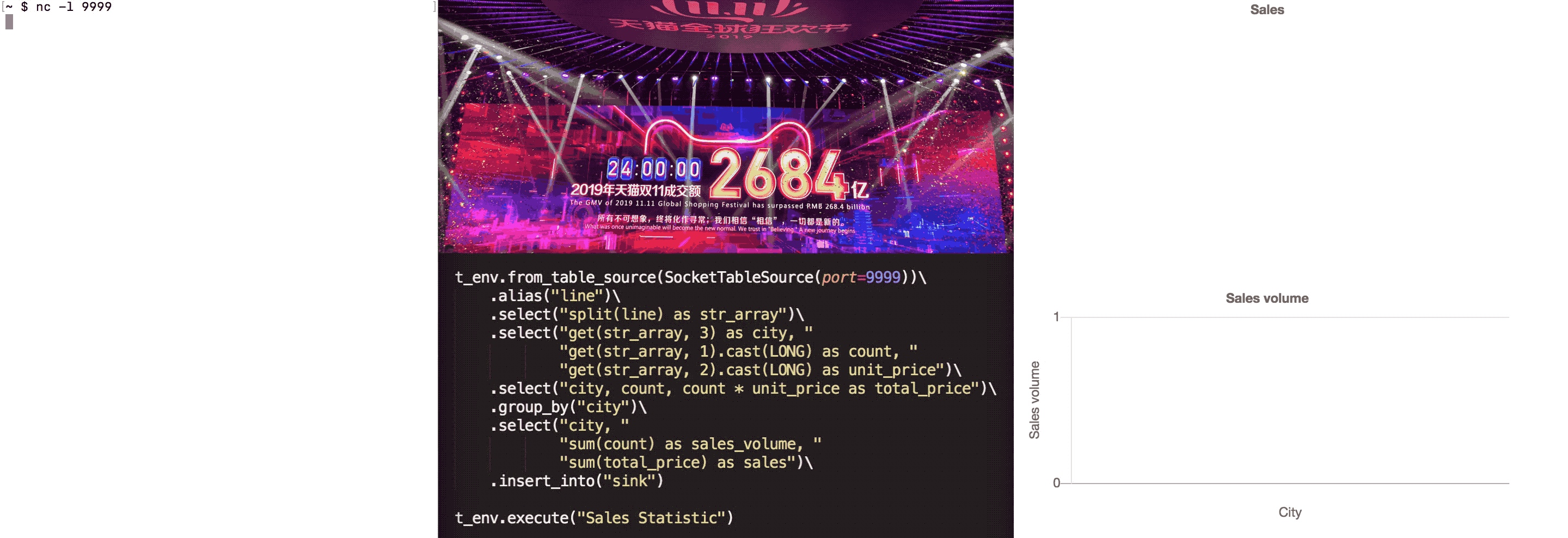## 总结和未来规划691 01576 0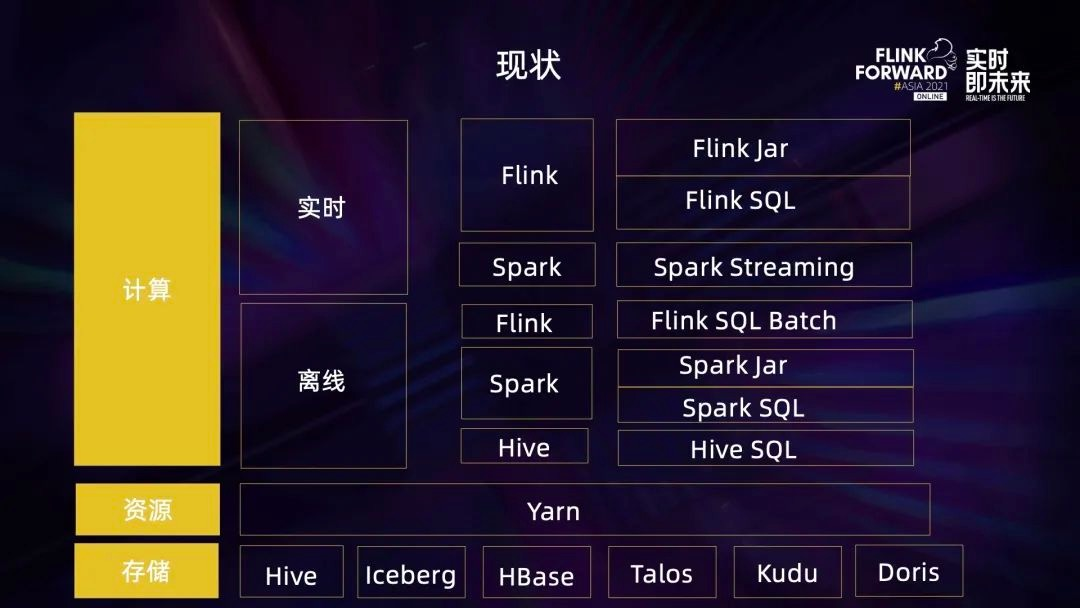66 0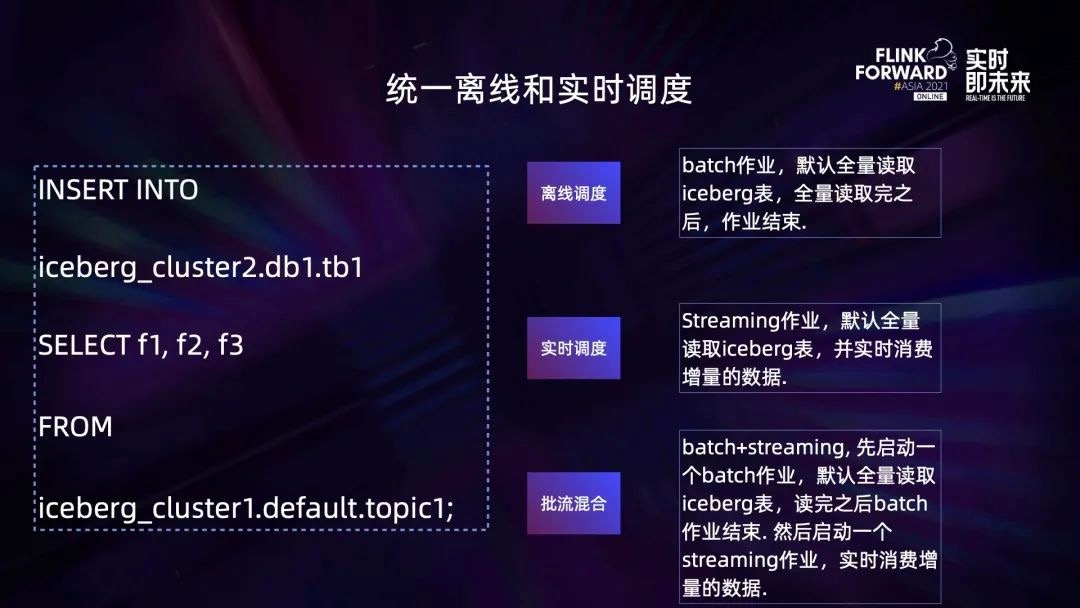45 0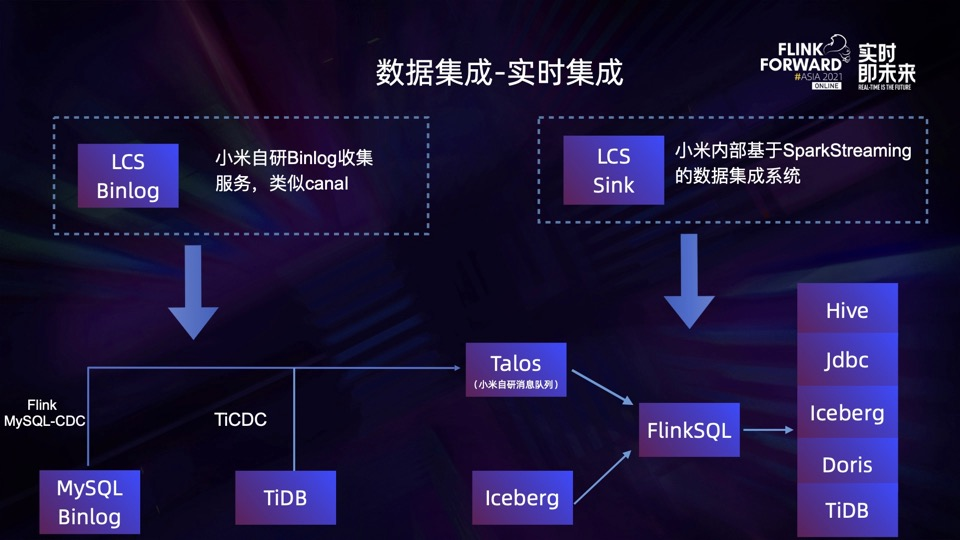60 051 033 0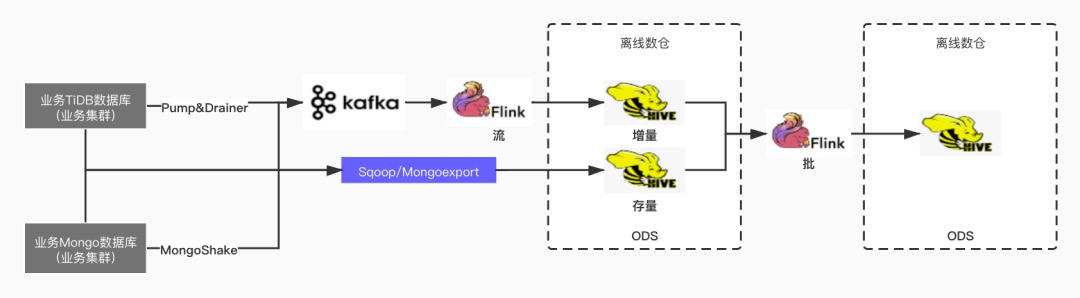45 0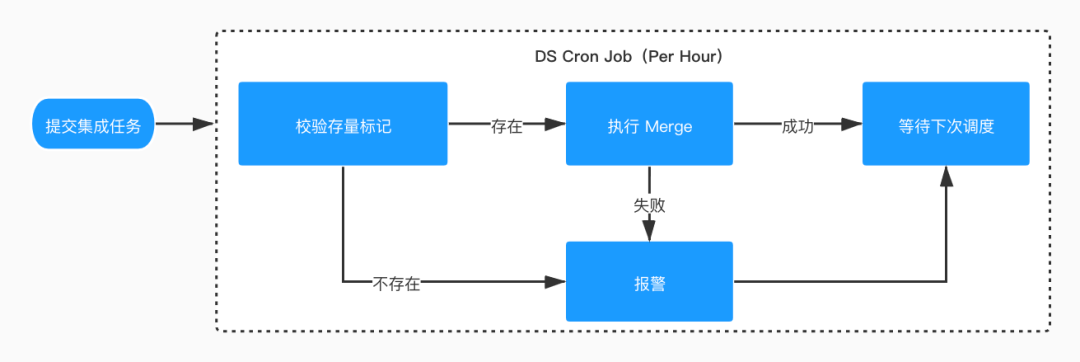41 0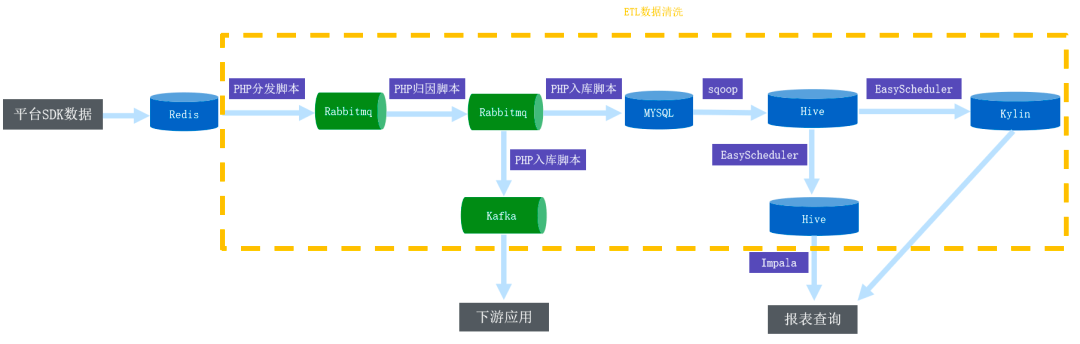55 0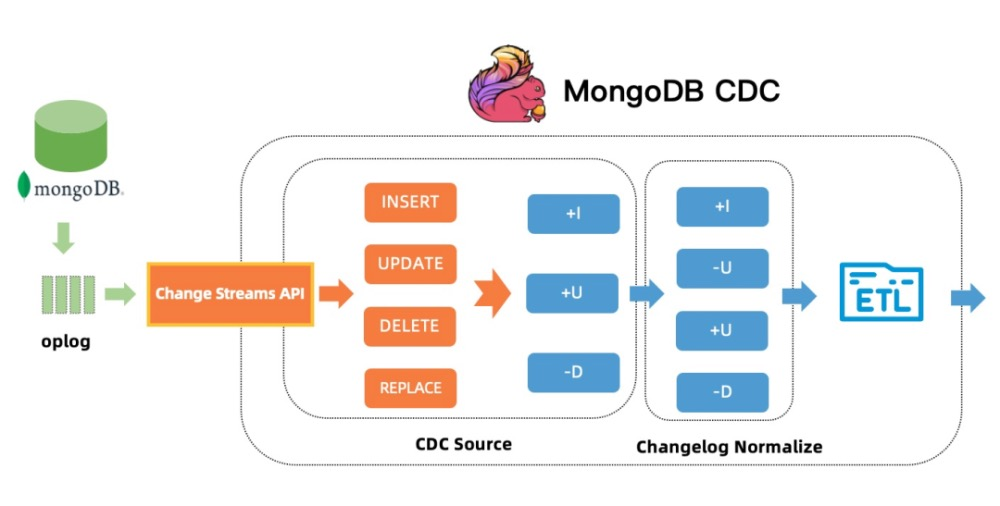28 033 0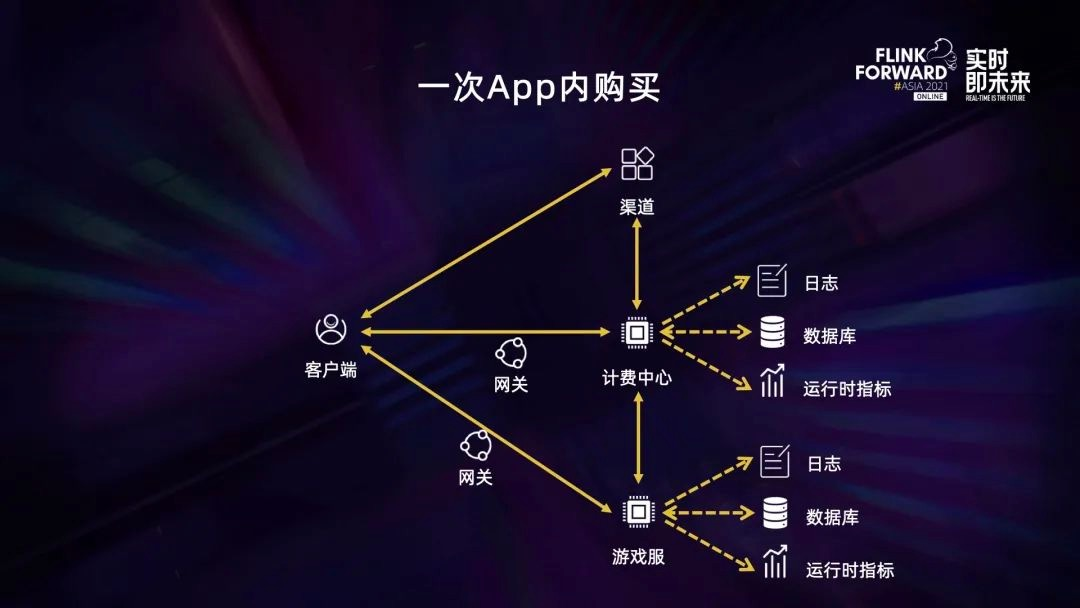29 0

【Python入门】Python数据处理编程基础3436

Apache NiFi Crash Course Intro